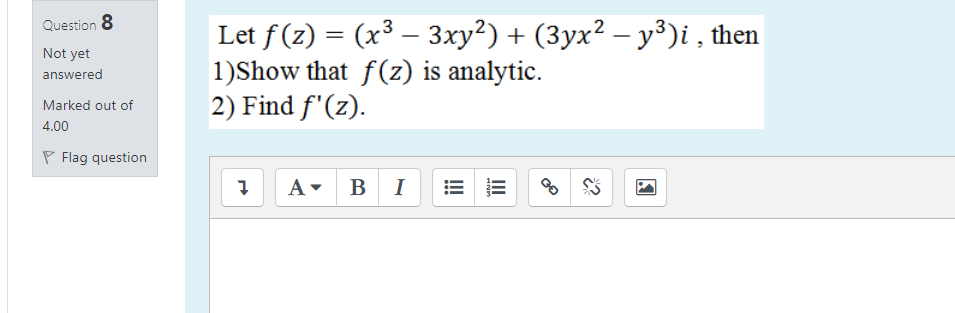### Create an Account

Already have account?

### Forgot Your Password ?

Home / Questions / Question 8 Not yet answered Let f(z) = (x3 – 3xy2) + (3yx2 - y3)i , then 1)Show that f(z) ...

# Question 8 Not yet answered Let f(z) = (x3 – 3xy2) + (3yx2 - y3)i , then 1)Show that f(z) is analytic. 2) Find f'(z). Marked out of 4.00 Flag question 7 A B 1 c?

Question 8 Not yet answered Let f(z) = (x3 – 3xy2) + (3yx2 - y3)i , then 1)Show that f(z) is analytic. 2) Find f'(z). Marked out of 4.00 Flag question 7 A B 1 c?Apr 13 2021 View more View Less

#### Answer (Solved)Subscribe To Get Solution